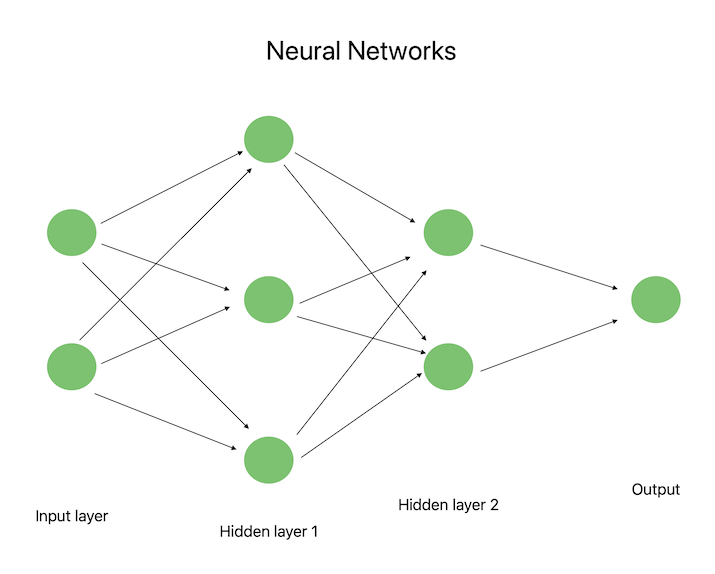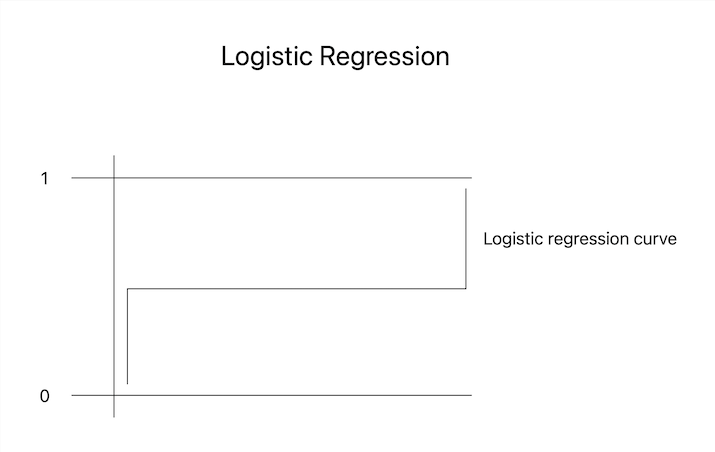# Difference Between Neural Network and Logistic Regression

Neural networks and logistic regression are significant machine learning technologies that help solve a variety of classification and regression problems. These models have gained popularity as a result of their precision in making predictions and their adaptability in processing various kinds of data. Neural networks, for instance, are useful in fields like picture identification and natural language processing because they can recognize patterns in data that are difficult to see and capture non-linear correlations in data. On the other hand, since it is straightforward and simple to understand, binary outcome situations frequently benefit from using logistic regression. In addition, more sophisticated models like neural networks can be built on the foundation of logistic regression. Machine learning practitioners must completely comprehend the distinctions between these models and their applications in order to choose the appropriate algorithm for their specific problem and get the best results. In this post, we will closely look at neural networks and logistic regression and the differences between them.

## What is a Neural Network?

A neural network is a machine-learning model based on the anatomy of the human brain. Information is processed and sent by layers of networked neurons. Each neuron receives input from the layer before it processes it using a non-linear activation function, and then sends its output to the layer after it. The model's anticipated value is represented by the output of the last layer. In domains including image identification, audio recognition, and natural language processing, neural networks are particularly useful for both regression and classification problems. Neural networks are a common solution for hard machine learning challenges because of their capacity to recognize patterns in data and capture non-linear correlations.## What is Logistic Regression?

In machine learning, logistic regression, a subset of linear regression, is used to determine the likelihood of a binary outcome. In this statistical model, the result of linear regression is converted into a number between 0 and 1, which denotes the chance that an event will occur. In fields including banking, healthcare, and marketing, logistic regression is frequently used since it is straightforward and basic. It is suitable for binary classification issues with true/false, 0/1, or yes/no outcomes. In logistic regression, maximum likelihood estimation is used to estimate the model's parameters, and regularization methods like L1 and L2 are typically applied to lessen overfitting.## Difference Between Neural Network and Logistic Regression

Here are the key differences between neural network and logistic regression −

Neural Network

Logistic Regression

It is a sophisticated and adaptable machine-learning strategy.

It is a straightforward and understandable algorithm.

It can be applied to both classification and regression problems.

It is appropriate for issues involving binary categorization.

Designed after the anatomy of the human brain

The results of linear regression are transformed using a logistic function.

There are several layers of linked neurons involved.

It presupposes that the dependent and independent variables have linear connections.

It can recognize patterns in data and capture non-linear relationships.

It can only simulate linear decision boundaries.

It takes substantially more memory and processing power.

It uses less processing power and memory.

It can deal with complicated data and numerous input variables.

Only binary input variables are permitted.

For complicated problems, it can generate forecasts that are more accurate.

If there are issues with non-linear decision boundaries, it might not be appropriate.

Implementation and training might be difficult.

Simple to use and train

It can need regularization and be prone to overfitting.

It may not need regularization and is less prone to overfitting.

## Conclusion

In conclusion, logistic regression, and neural networks are two independent machine learning techniques, each with particular benefits and drawbacks. While more complex and able to capture non-linear interactions, neural networks nevertheless need more computer resources and can be more challenging to build and train. In contrast, logistic regression is clear and easy to grasp, but it is limited to binary input variables and linear decision constraints. Understanding the differences between these models is essential to selecting the optimal algorithm for a particular problem and achieving the best outcomes.

Updated on: 25-Apr-2023

391 Views